Graphs & Tables
Independent vs. Dependent Variables
Random Math
EXTRA
100

What is the ordered pair of the triangle?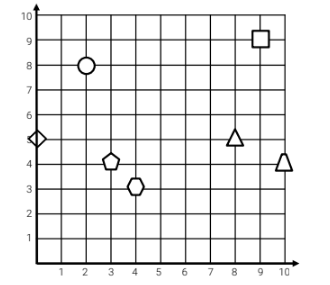(8,5)

100

Is the following example a multiplicative or additive relationship?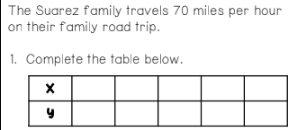Multiplicative Relationship

100

Which is the independent variable and which is the dependent variable?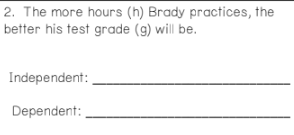IV: hours practiced

100

Is this relationship multiplicative? Additive? or Neither?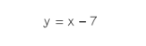100

Mulan

200

Plot the point (5,20)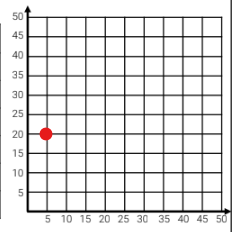200

Write an equation for the example below... (it's additive)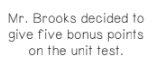y=x+5

200

What is the Independent and Dependent variables?IV: How many months subscribed

DV: Monthly Cost

200

Write an equation for the graph below...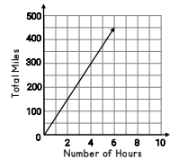y=75x

200

Drake and Josh

300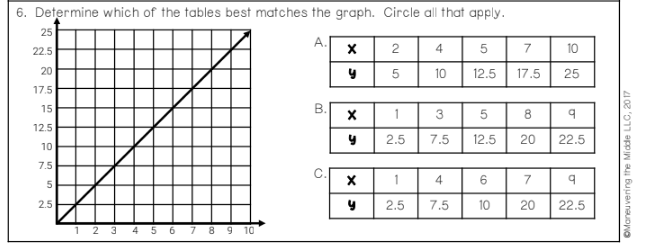A & B

300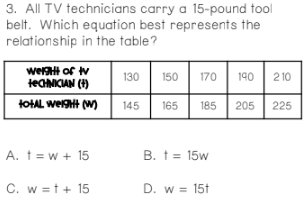C

300

What is the IV and DV?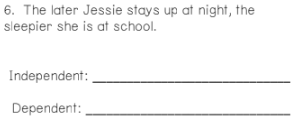IV: How late Jesse stays up

DV: How tired she is

300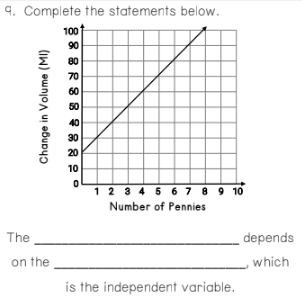Change in volume depends on the number of pennies

300
Who was the 2020-2021 NFL MVP

Aaron Rodgers

400

Complete the table with the graph below.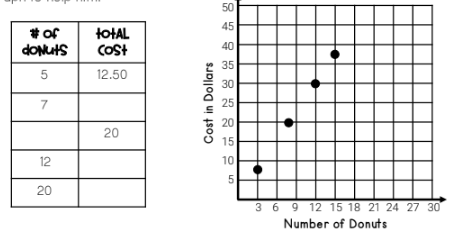What is multiplying by 2.5

400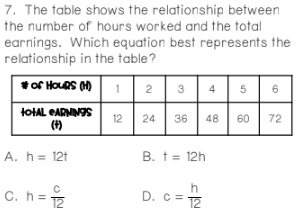B

400

What are the dependent quantities of the graph below...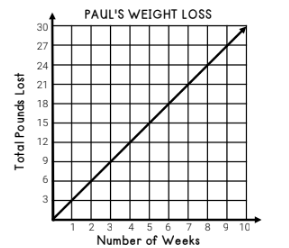3, 6, 9, 12, 15, 18, 21, 24, 27, 30

400

Is this a multiplicative or additive relationship?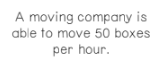multiplicative

400

Who has the most followers on Instagram. Name 1 in the top 5.500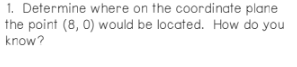On the X axis

500

Write an equation for the graph below.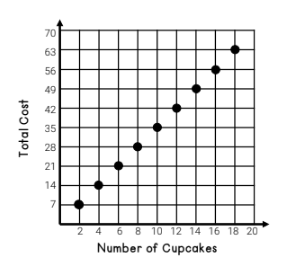y=3.5x or c=3.5t

500

What is the independent variable of the example below...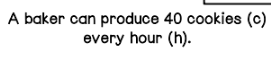Hours

500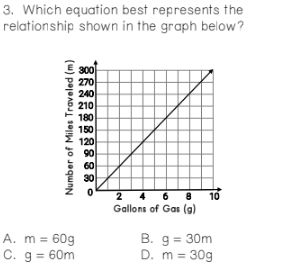D

500

What was the most watched series streamed on netflix in 2020?Click to zoom